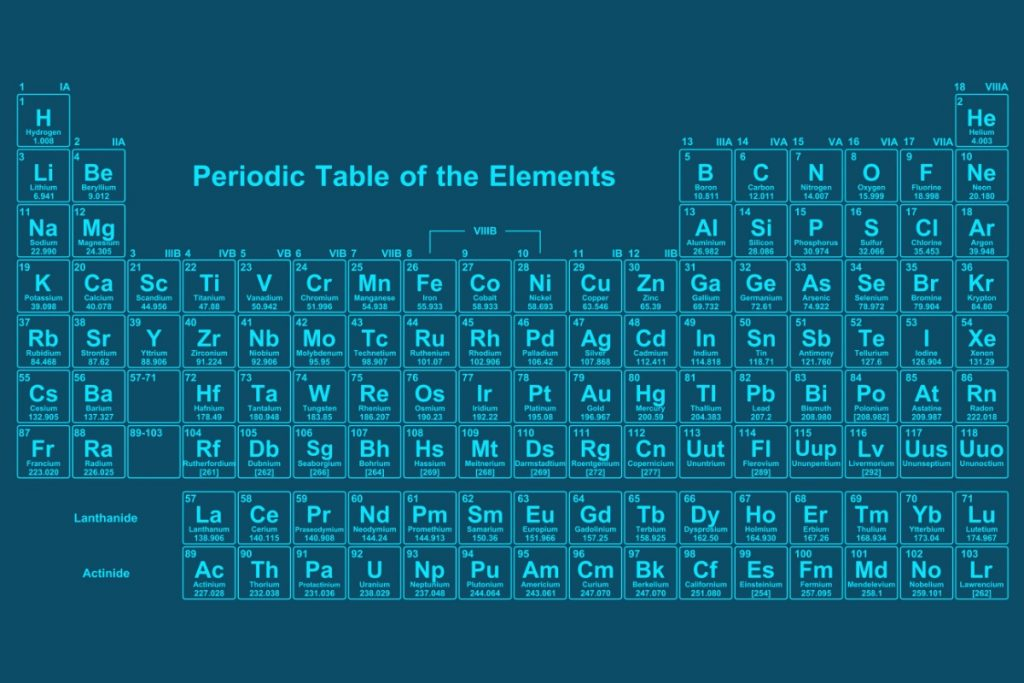Select Page

## Here we will look at the key things to remember, mistakes to avoid and also show example equations we think would be useful to learn and be able to balance.

### Symbols

You should now be familiar with the periodic table showing you all the chemical elements and their symbols. There are some symbols you will learn instantly and remember and some compounds you will know; for example, students usually recall the formula for water (H₂O) and carbon dioxide (CO₂). It is important you can also write these correctly. Pay attention to whether the element has just one letter or two. For example, carbon is C and oxygen is O, but sodium is Na and magnesium is Mg. It is not as simple as using the first letter of the element to fit the symbol, even though sometimes this is the case. If the element has two letters as part of the symbol, such as magnesium, the first is always capital and then the second is lower case (Mg), the same for Helium (He).### Compounds

When writing the formula for compounds, remember that as they are a new substance created from a reaction of two or more elements then these contain capital and lowercase letters. Magnesium oxide is formed as a result of the reaction between Magnesium and Oxygen. The formula for magnesium oxide is MgO. For carbon dioxide the chemical formula is CO₂, it is important that the number is subscript, small and at the bottom of the O.

### Chemical Equations

There are some chemical equations you are expected to learn and know and some are easier than others. In previous blogs, we have talked about the equations for respiration and photosynthesis. There are also some simple chemical reactions you will learn; those for combustion of materials, acid reactions, and electrolysis are just a few examples. When writing these you need to know the names and be careful of the spellings for each element and compound. You need to be clear if you are being asked to write a word equation or a symbol equation. NEVER write a combination of the two, stick to one or the other.

### Balancing Equations

As you learn more and progress through KS3 and then KS4 Science you will learn to write and balance symbol equations. Often these are simple reactions such as burning a chemical in oxygen. For example the reaction of magnesium with oxygen:

Magnesium + Oxygen → Magnesium Oxide

The symbol equation for this reaction is:

MgO + O₂ → MgO

You then need to check there are equal numbers of each type of atom for the reactants and products.

For this equation, there is 1 Magnesium atom and 3 Oxygen atoms reacting to form Magnesium Oxide which has 1 magnesium atom and 1 oxygen atom, so we need to balance this out. To do this you are allowed to balance the number of atoms on each side so we need a 2 in front of the magnesium and a 2 in front of the magnesium oxide:

2MgO + O₂ → 2MgO

You have now got two magnesium and two oxygen atoms on each side.

Hopefully, this gives you some help on how to be confident in writing chemical formulas for elements and compounds.

Remember:

🧪 You cannot change the letters, you need to be careful with your capitals and lowercase letters
🧪 You can only add numbers in the front of elements or compounds to balance a symbol equation
🧪 In a compound the numbers that come after a letter are always subscript; never to be written like a squared number or full size:

CO2 ❌
CO² ❌
CO₂ ✅